# cockrum.net

## Code

### Example of Counting Zeros and Ones from the TrueRNGpro

On a machine that has a functional TrueRNG install, Python 2.7 or 3.8+, and Pyserial, you just need to download the python code (count_bits.py) and run it from the directory where you put it with:

``python count_bits.py``

Python is available here if you need it.

This is what the output looks like when capturing with a TrueRNGpro. There is a 1,048,576 byte "random.bin" file in the current directory that conatains the captured data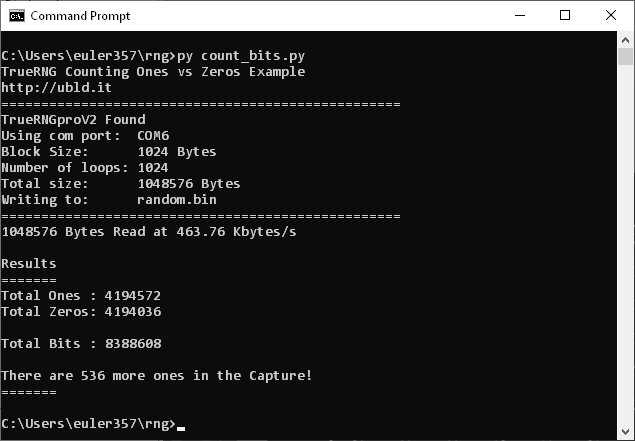You can change blocksize or numloops to capture different amounts of data or modify the code for your own purposes

Here's the Python code listed below.

``````
#!/usr/bin/python

# TrueRNG Read - Simple Example
# Chris K Cockrum
# 7/9/2021
#
# Requires Python 2.7 or 3.7+, pyserial
# On Linux - may need to be root or set /dev/tty port permissions to 666
#
# Python available here: https://www.python.org/
# Install Pyserial package with:   python -m pip install pyserial

import serial
import time
import os
from serial.tools import list_ports

# Number of Bytes to Capture per Block
blocksize=1024

# Number of Blocks to Capture
numloops=1024

print('TrueRNG Counting Ones vs Zeros Example')
print('http://ubld.it')
print('==================================================')

import serial.tools.list_ports

# ubld.it TrueRNG
TrueRNGpid="04D8"
TrueRNGhid="F5FE"

# ubld.it TrueRNGpro
TrueRNGpropid="16D0"
TrueRNGprohid="0AA0"

# ubld.it TrueRNGproV2
TrueRNGproV2pid="04D8"
TrueRNGproV2hid="EBB5"

# Set default of None for com port
rng_com_port = None

ports = list(serial.tools.list_ports.comports())

for p in ports:
if(rng_com_port == None):
if TrueRNGproV2pid and TrueRNGproV2hid in p.hwid:
rng_com_port = p.device
print('TrueRNGproV2 Found')
break
if TrueRNGpropid and TrueRNGprohid in p.hwid:
rng_com_port = p.device
print('TrueRNGpro Found')
break
if TrueRNGpid and TrueRNGhid in p.hwid:
rng_com_port = p.device
print('TrueRNG Found')
break

if rng_com_port == None:
exit()

# Print which port we're using
print('Using com port:  ' + str(rng_com_port))

# Print block size and number of loops
print('Block Size:      ' + str(blocksize) + ' Bytes')
print('Number of loops: ' + str(numloops))
print('Total size:      ' + str(blocksize * numloops) + ' Bytes')
print('Writing to:      random.bin')
print('==================================================')

# Open/create the file random.bin in the current directory with 'write binary'
fp=open('random.bin','wb')

# Print an error if we can't open the file
if fp==None:
print('Error Opening File!')

# Try to setup and open the comport
try:
ser = serial.Serial(port=rng_com_port,timeout=10)  # timeout set at 10 seconds in case the read fails
except:
print('Port Not Usable!')
print('Do you have permissions set to read ' + rng_com_port + ' ?')

# Open the serial port if it isn't open
if(ser.isOpen() == False):
ser.open()

# Set Data Terminal Ready to start flow
ser.setDTR(True)

# This clears the receive buffer so we aren't using buffered data
ser.flushInput()

# Keep track of total bytes read
totalbytes=0
totalzeros=0

# Generate look-up table for number of 1's in a byte
ones_in_byte =  * 256
for n in range(256):
ones_in_byte[n] = bin(n).count("1")

totalones=0
totalzeros=0

starttime=time.time()
# Loop
for _ in range(numloops):

# Try to read the port and record the time before and after
try:
except:
break

totalbytes +=len(x)

# Count ones
for n in range(len(x)):
totalones=totalones + ones_in_byte[x[n]]
totalzeros=totalzeros + 8-ones_in_byte[x[n]]

# If we were able to open the file, write to disk
if fp !=0:
fp.write(x)

# Calculate the rate
timenow=time.time()
if(timenow!=starttime):
rate=float(totalbytes) / ((timenow-starttime)*1000.0)

print(str(totalbytes) + ' Bytes Read at ' + '{:6.2f}'.format(rate) + ' Kbytes/s',end='\r')

print('\n\nResults')
print(    '=======')
print('Total Ones : ' + str(totalones))
print('Total Zeros: ' + str(totalzeros) + '\n')
print('Total Bits : ' + str(totalbytes*8))

if (totalones==totalzeros):
print('\nEqual Number of Ones and Zeros in Capture!')
if (totalones>totalzeros):
print('\nThere are ' + str(totalones-totalzeros) + ' more ones in the Capture!')
else:
print('\nThere are ' + str(totalzeros-totalones) + ' more zeros in the Capture!')

print(    '=======')

# Close the serial port
ser.close()

# If the file is open then close it
if fp != 0:
fp.close()

# If we're on Linux set min on com port back to 1
# Pyserial screws this up
if os.name == 'posix':
os.system('stty -F '+rng_com_port+' min 1')

``````

## New TrueRNG Utilities

I have written and tested a set of Python utilities for TrueRNG devices

### Tools (Windows and Linux)

• truerng_find.py: Scans COM ports and identifies all connected TrueRNG devices
• truerng_mode.py: Example of how to change modes on TrueRNGpro and TrueRNGproV2 devices
• truerng_test.py: Finds and performs testing on connected TrueRNG devices

### Tools (Linux Only)

• truerng_fulltest.py: Reads a large block of data (14GB) and runs ent, rngtest, and dieharder (takes ~9 hours on the TrueRNGpro / TrueRNGproV2)
• run_rngtest: Linux script to run rngtest since it doensn't like to be called directly from Python - this is a "helper" for truerng_fulltest.py and isn't meant to be used directly

### Example Output of truerng_test.py on TrueRNG V1/V2/V3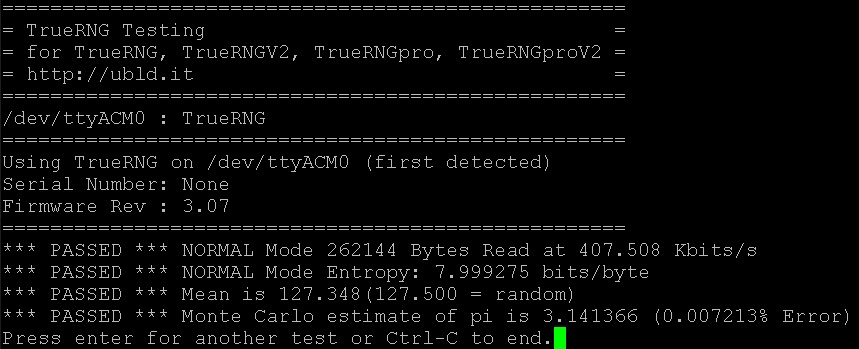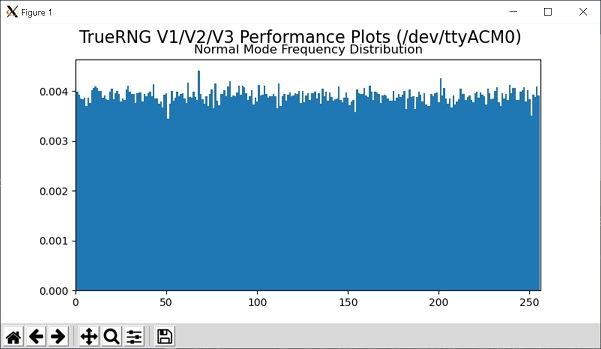### Example Output of truerng_test.py on TrueRNGpro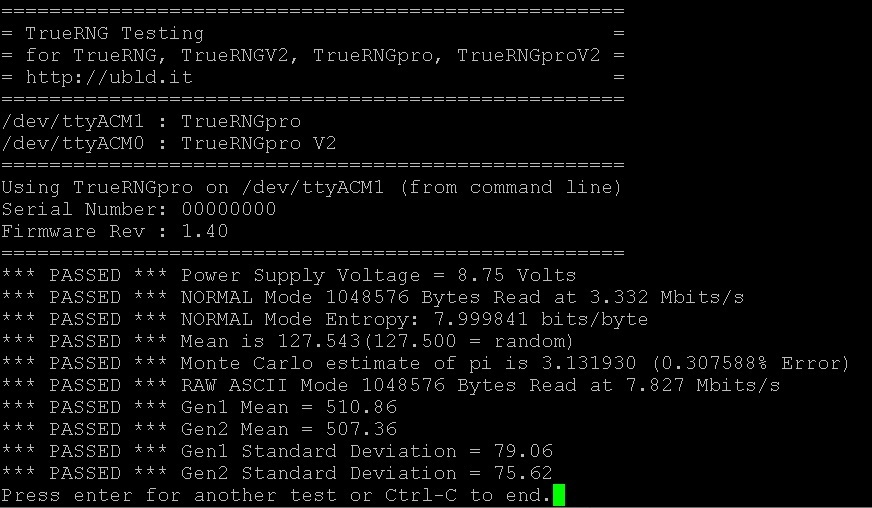## How to Convert a Uniform Distribution to a Gaussian Distribution

The TrueRNGv2/3 and many other random number generators often provide a uniform distribution output. This means that you are getting a binary file where each bit has an equal probability of being a zero or one.

If you take 8 bits at a time, then you have a 1 in 256 (2^8) chance that this 8-bit byte will be a particular value. If you take 32 bits at a time, then you have a 1 in 4,294,967,294 (2^32) chance that this 32-bit word will be a particular value.

When plotted as a histogram, a uniform distribution looks like this: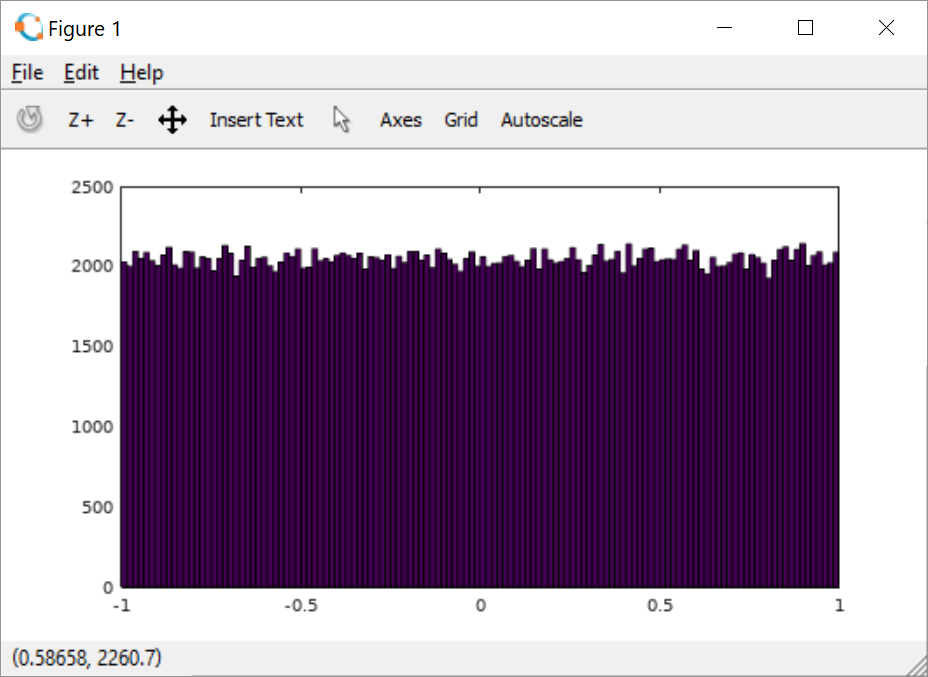Many natural random processes produce a Gaussian distribution (i.e. the "bell curve" shape) and this distribution is used for many different applications.

When plotted as a histogram, a Gaussian distribution looks like this:Since we get questions about how to get different distributions, I wrote the below C program to do this. It uses the Box-Muller transform which has a great explanation on Wikipedia so I won't describe it here.

This was written and tested on an Ubuntu 16.04 x86-64 Linux system but should be easily convertable to other platforms

To compile this, you need the basic build-essential package (sudo apt install build-essential) and the files in the zip below

Unzip them to a directory, then type "Make" - this should produce the ugdist executable file

To capture data from the TrueRNG/TrueRNGpro, you just need to use dd to get a file like this:

``dd if=/dev/TrueRNG of=./uniform_file bs=1024 count=1024 iflag=fullblock``

This will produce a 1MiB file (1024 byte blocks x 1024 count) called uniform_file

To change this to a Gaussian distribution, use the ugdist tool:

``/ugdist ./uniform_file ./gaussian_file``

This will quickly produce an ASCII formatted output of floating point numbers that are Gaussian distributed with the mean and standard deviation defined in the ugdist.c file

The output file can be loaded into Octave (or Matlab) and display a histogram using this command where "100" is the number of equally sized bins:Here's a zip of the C code below and the associated Makefile.

Here's a 1MiB binary file of uniform distributed numbers.

Here's the same file converted to ASCII floats and normalized.

Here's the file converted to a Gaussian distribution saved as ASCII floats (mean=0, standard dev=10).

``````
/****************************************************
* Uniform to Gaussian Distribution                  *
* Uses Box-Muller transform                         *
* Ref: http://bit.ly/2GIs0mn                        *
*                                                   *
* Chris K Cockrum                                   *
* https://cockrum.net                               *
* 02/03/2018                                        *
*                                                   *
* Infile: binary uniform distribution file          *
* (i.e. from TrueRNG binary mode capture)           *
* OutFile: ASCII text file of floating point values *
* Output will have Gaussian distribution with mean  *
* and standard deviation as defined below or input  *
* conversion if the INPUT_ONLY is set               *
****************************************************/

#include
#include

#define two_pi    2*3.14159265358979323846264338327
#define mean      0.0
#define stddev    10.0

#define INPUT_ONLY 1

int main(int argc, char** argv)
{
FILE *infile;           /* Input File Pointer */
FILE *outfile;          /* Output File Pointer */
FILE *outfile2;         /* Output File Pointer */
int x_in,y_in;          /* Variables to read input binary values */
double x,y;             /* Variables for input values as floats */
double g0,g1;           /* Variables to hold Gaussian distributed values */

/* If we don't have 2 command line inputs */
if (argc !=3 )
{
printf("Usage:\n ugdist infile outfile\n");
printf("Infile: binary uniform distribution file (i.e. from TrueRNG binary mode capture)\n");
printf("OutFile: ASCII text file of floating point values\n");
return 0;
}

/* Open the Input File */
infile=fopen(argv,"rb");
if(infile<=0)
{
printf("Can't open %s\n",argv);
return 0;
}

/* Open the Output File */
outfile=fopen(argv,"w+");
if(outfile<=0)
{
printf("Can't open %s\n",argv);
fclose(infile);
return 0;
}

/* Print output file comment */
fprintf(outfile,"%% Gaussian Distribution Random Numbers\n");

while(1)
{
/* Read 4 bytes to each int */

/* If we are out of data then exit this loop */
break;

/* Scale to +/- 1 */
x=(double)x_in / (double)(1<<31);
y=(double)y_in / (double)(1<<31);

#if INPUT_ONLY

/* Only print converted and scaled input values */
fprintf(outfile,"%f\n",x);
fprintf(outfile,"%f\n",y);

#else

/* If x != 0 -- prevent discontinuity in log at 0 */
if(x!=0)
{

/* change sign for -log since the log function doesn't support complex numbers */
if(x>0)
{
g0=sqrt(-2.0*log(x))*cos(two_pi*y);
g1=sqrt(-2.0*log(x))*sin(two_pi*y);
}
else
{
g0=-sqrt(-2.0*log(-x))*cos(two_pi*y);
g1=-sqrt(-2.0*log(-x))*sin(two_pi*y);
}

/* Apply mean and standard deviation then print to output file */
fprintf(outfile,"%f\n",g0*stddev+mean);
fprintf(outfile,"%f\n",g1*stddev+mean);
}

#endif

};

/* Close Files */
fclose(infile);
fclose(outfile);

return 0;
}
``````

## A Simple Python TrueRNG Server Example

We've gotten a few questions about using the TrueRNG as a random number server so I banged out this quick example. I have tested this on Windows 10 and Ubuntu 16.04.01 (x64) using Python 2.7. This doesn't include any encryption which may be required for a particular application. There are many tutorials about how to encrypt data in python such as Laurent Luce's Blog

Here is a screenshot of the server after receiving 3 requests for random blocksHere is a screenshot of the client after running 3 times and receiving 64 byte ASCII hex random number stringsHere's a zip of the Python code below.

This is the server code. It receives a UDP packet on the port and IP specified and compares the date to the 'get random' string. If there is a match, it grabs 64 bytes from the TrueRNG and formats it into an ASCII encoded HEX string and sends it back to the originating IP address

``````
#!/usr/bin/python

# TrueRNG Read - Simple Random Number Server Example - Server Side
# Chris K Cockrum
# 8/26/2016
#
# Requires Python 2.7, pyserial
# On Linux - may need to be root or set /dev/tty port permissions to 666
#
# Python 2.7.xx is available here: https://www.python.org/
# Install Pyserial package with:   python -m pip install pyserial

import socket
import serial
import binascii
from serial.tools import list_ports

# Number of bytes to return
blocksize=64

# Port to listen on
listenport=5555;

#IP = '192.168.1.207'
IP = 'localhost'

print('TrueRNG Server Example')
print('http://ubld.it')
print('==================================================')

# Create ports variable as dictionary
ports=dict()

# Call list_ports to get com port info
ports_avaiable = list(list_ports.comports())

# Set default of None for com port
rng_com_port = None

# Loop on all available ports to find TrueRNG
for temp in ports_avaiable:
if temp.startswith("TrueRNG"):
print('Found:             ' + str(temp))
if rng_com_port == None:        # always chooses the 1st TrueRNG found
rng_com_port=str(temp)

# Print which port we're using
print('Using com port:    ' + str(rng_com_port))

# Print block size and number of loops
print('Block Size:        ' + str(blocksize) + ' Bytes')
print('==================================================')

# Try to setup and open the comport
try:
ser = serial.Serial(port=rng_com_port,timeout=10)  # timeout set at 10 seconds in case the read fails
except:
print('Port Not Usable!')
print('Is a TrueRNG installed?')
print('Do you have permissions set to read ' + rng_com_port + ' ?')

# Open the serial port if it isn't open
if(ser.isOpen() == False):
ser.open()

# Set Data Terminal Ready to start flow
ser.setDTR(True)

# This clears the receive buffer so we aren't using buffered data
ser.flushInput()

# Keep track of total bytes read
totalbytes=0

# Create a TCP/IP socket
sock = socket.socket(socket.AF_INET, socket.SOCK_DGRAM)

# Bind the socket to the port

try:
while True:

# Wait for connection and get data

# If we received the correct command
if data == 'get random':

# Read bytes from serial port and convert to an ASCII hex string

# Send data back to requestor
print('Sent %s characters (%s Random bytes) to %s' % (sent, str(blocksize), address))

finally:

# Close the socket
sock.close()

# Close the serial port
ser.close()
``````

This is the client code. It sends a UDP packet on the port and IP specified and then waits for a response on the same port and IP. It then prints the response

``````
#!/usr/bin/python

# TrueRNG Read - Simple Random Number Server Example - Client Side
# Chris K Cockrum
# 8/26/2016
#
# Requires Python 2.7, pyserial
# On Linux - may need to be root or set /dev/tty port permissions to 666
#
# Python 2.7.xx is available here: https://www.python.org/
# Install Pyserial package with:   python -m pip install pyserial

import socket
import sys
import errno

# Port
port = 5555

#IP = '192.168.1.207'
IP = 'localhost'

try:
# Create a UDP socket
sock = socket.socket(socket.AF_INET, socket.SOCK_DGRAM)

# Set a timeout to wait (2 seconds)
sock.settimeout(2.0)

message = 'get random'

# Send data

data, server = sock.recvfrom(4096)

except socket.error, v:
print('Error: ' + str(v))

finally:
sock.close()
``````

## Step-by-Step: Using TrueRNGpro with Python in Windows 10 and Linux

We get questions about how to use our TrueRNG random number generators so I thought I would write a tutorial on how to get it running with Python on Windows and Linux

### Installing the TrueRNG & Driver

On Windows, you download and install the .inf file then plug in the device. You should see it come up in the device manager as a 'Port' (Window-x on the keyboard, then click Device Manager in Windows 10)On Linux, you should see the device in your dmesg (i.e. "dmesg | tail"). Installing the udev rule per the instructions will make it show up also as /dev/TrueRNG.Note: on Linux, to access the TrueRNG as a user, you may need to modify the device node permissions to allow users to access the hardware directly. You can use the below command for this.

``sudo chmod 666 /dev/TrueRNG``

or change to whatever device node that your system assigns to the device like:

``sudo chmod 666 /dev/ttyACM0``

### Installing Python 2.7 and pyserial on Windows 10

On Windows, you go to the Python website and download the "Windows x86-64 MSI installer" or "Windows x86 MSI installer" for the latest version of Python 2.7 (currenly it is 2.7.12). Run the install program and install it with all of the default options. This should install Python in c:\python\python2.7 or c:\python2.7

Next, we need to add the Python directories to the path. To get there in Windows 10, you use Window-x, click "system", click "Advanced system settings", then click "Environement Variables". Choose the "Path" variable and click edit. You should add your Python27 and Python27\Scripts directories here according to the install directory you chose.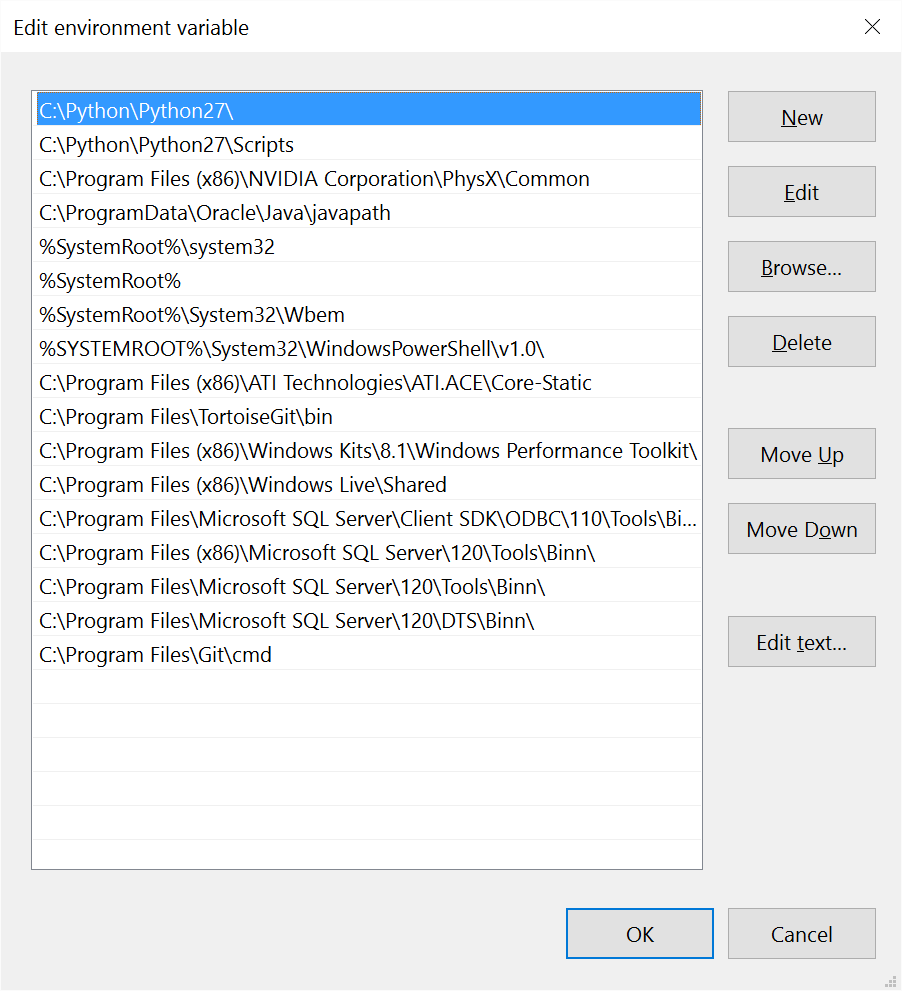To install pyserial (Python serial port library), open up a Command Prompt window and use "python -m pip install pyserial" to install it. If this doesn't work then your environment variables aren't correct or you need to open up a new Command Prompt window to use the new variables.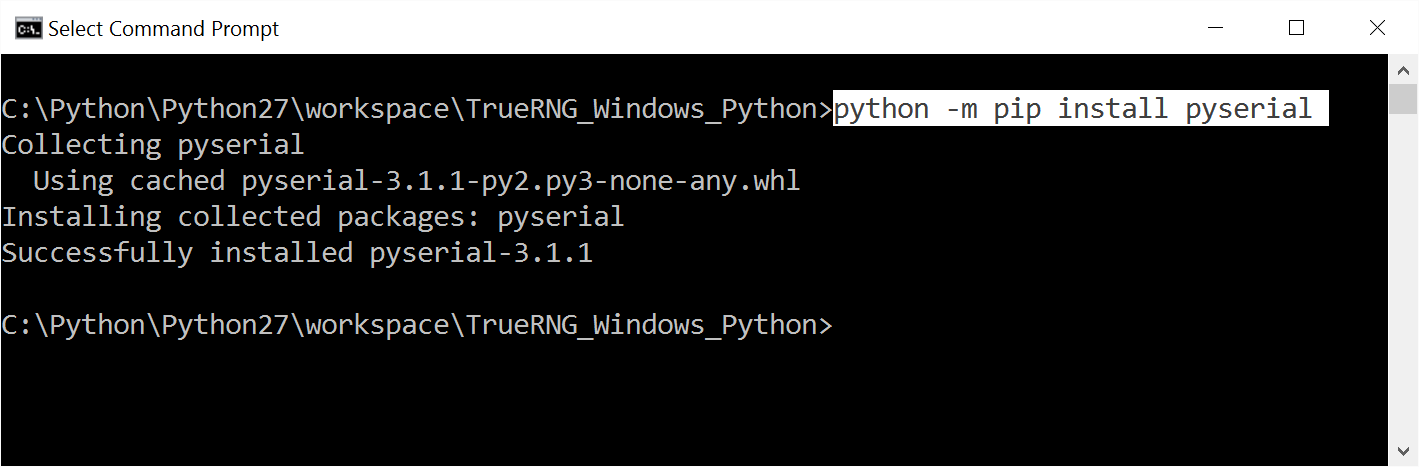To test the install, run "python" from a Command Prompt window and then try "import serial". If this work with no errors, then you should be good with the Python and pyserial install.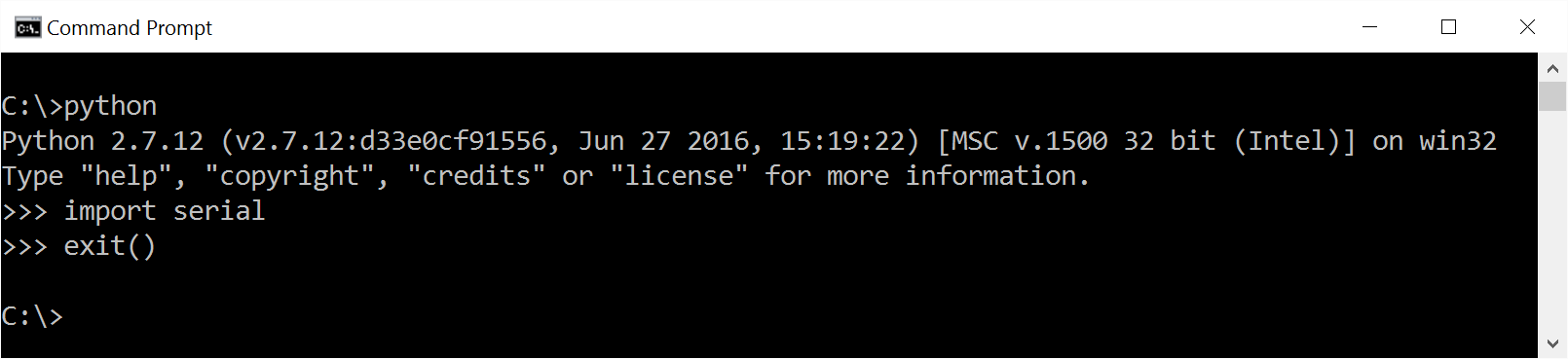### Installing Python 2.7 and pyserial on Ubuntu Linux

For Ubuntu (or other Linuxes that use apt), log in and issue the commands:

``sudo apt-get update``
``sudo apt-get install python2.7``
``sudo apt-get install python-pip``
``python -m pip install pyserial``

### Running the example code

On a machine that has a functional TrueRNG install, Python 2.7, and Pyserial, you just need to download the python code (read_truerng_example.py) and run it from the directory where you put it with:

``python read_truerng_example.py``

This is what the output looks like when capturing with a TrueRNGpro. There is a 1,024,000 byte "random.bin" file in the current directory that conatains the captured data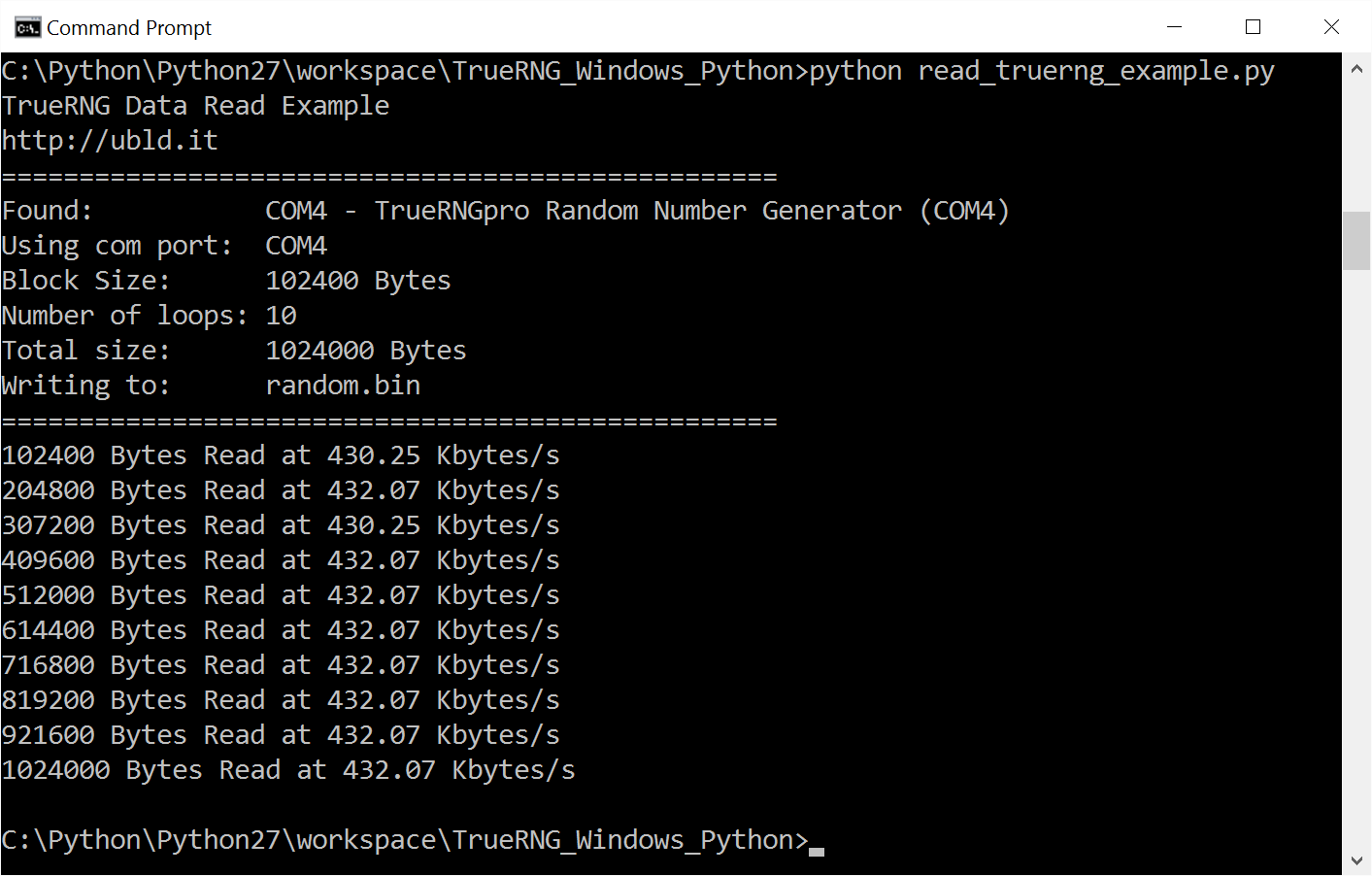You can change blocksize or numloops to capture different amounts of data or modify the code for your own purposes

Here's the Python code listed below.

``````
#!/usr/bin/python

# TrueRNG Read - Simple Example
# Chris K Cockrum
# 8/21/2016
#
# Requires Python 2.7, pyserial
# On Linux - may need to be root or set /dev/tty port permissions to 666
#
# Python 2.7.xx is available here: https://www.python.org/
# Install Pyserial package with:   python -m pip install pyserial

import serial
import time
from serial.tools import list_ports

# Size of block for each loop
blocksize=102400

# Number of loops
numloops=10

print('http://ubld.it')
print('==================================================')

# Create ports variable as dictionary
ports=dict()

# Call list_ports to get com port info
ports_avaiable = list(list_ports.comports())

# Set default of None for com port
rng_com_port = None

# Loop on all available ports to find TrueRNG
for temp in ports_avaiable:
if temp.startswith("TrueRNG"):
print('Found:           ' + str(temp))
if rng_com_port == None:        # always chooses the 1st TrueRNG found
rng_com_port=str(temp)

# Print which port we're using
print('Using com port:  ' + str(rng_com_port))

# Print block size and number of loops
print('Block Size:      ' + str(blocksize) + ' Bytes')
print('Number of loops: ' + str(numloops))
print('Total size:      ' + str(blocksize * numloops) + ' Bytes')
print('Writing to:      random.bin')
print('==================================================')

# Open/create the file random.bin in the current directory with 'write binary'
fp=open('random.bin','wb')

# Print an error if we can't open the file
if fp==None:
print('Error Opening File!')

# Try to setup and open the comport
try:
ser = serial.Serial(port=rng_com_port,timeout=10)  # timeout set at 10 seconds in case the read fails
except:
print('Port Not Usable!')
print('Do you have permissions set to read ' + rng_com_port + ' ?')

# Open the serial port if it isn't open
if(ser.isOpen() == False):
ser.open()

# Set Data Terminal Ready to start flow
ser.setDTR(True)

# This clears the receive buffer so we aren't using buffered data
ser.flushInput()

# Keep track of total bytes read
totalbytes=0

# Loop
for _ in range(numloops):

# Try to read the port and record the time before and after
try:
before = time.time()    # in microseconds
after = time.time()     # in microseconds
except:
break

totalbytes +=len(x)

# If we were able to open the file, write to disk
if fp !=0:
fp.write(x)

# Calculate the rate
rate=float(blocksize) / ((after-before)*1000.0)

print(str(totalbytes) + ' Bytes Read at ' + '{:6.2f}'.format(rate) + ' Kbytes/s')

# Close the serial port
ser.close()

# If the file is open then close it
if fp != 0:
fp.close()
``````

## If you need a hardware random number generator, take a look at http://TrueRNG.net and http://TrueRNGpro.com